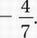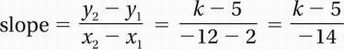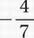# SAT Math Multiple Choice Question 776: Answer and Explanation

### Test Information

Question: 776

11. In the xy-plane, points A(2, 5) and B(-12, k) lie on a line that has a slope ofWhat is the value of k?

• A. 13
• B. 16.5
• C. 18
• D. 29.5

Explanation:

A

Algebra (graphs of linear equations) MEDIUM

Recall the slope formula from Chapter 7, Lesson 5:Since the slope equals:

Cross-multiply:

7k - 35 = 56